# June 3rd Electronics TV's & Laptops

### Auction Details

Electronics TV's & Laptops
Choose Your Layout >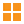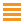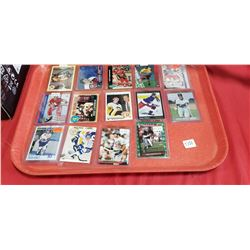151 - Shelf One
CDC Auctions
Shelf One
Bidding Has Concluded

Sold to f***r for (10.00 + 1.30) x 1 = 11.30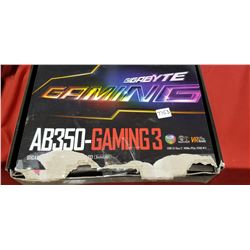153 - Shelf One
CDC Auctions
Shelf One
Bidding Has Concluded

Sold to f***r for (25.00 + 3.25) x 1 = 28.25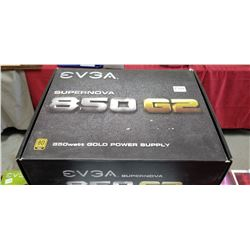154 - Shelf One
CDC Auctions
Shelf One
Bidding Has Concluded

Sold to f***r for (75.00 + 9.75) x 1 = 84.75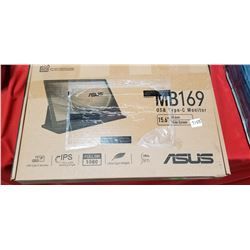155 - Shelf One
CDC Auctions
Shelf One
Bidding Has Concluded

Sold to S********r.. for (75.00 + 9.75) x 1 = 84.75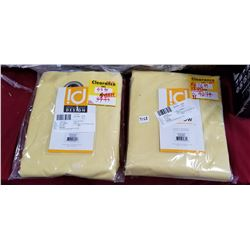158 - Shelf One
CDC Auctions
Shelf One
Bidding Has Concluded

Sold to f***r for (10.00 + 1.30) x 1 = 11.30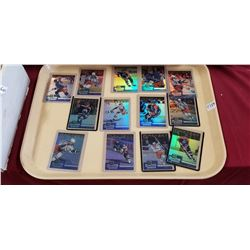159 - Shelf One
CDC Auctions
Shelf One
Bidding Has Concluded

Sold to k********z for (12.50 + 1.63) x 1 = 14.13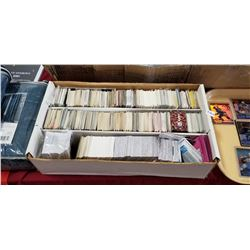160 - Shelf Two
CDC Auctions
Shelf Two
Bidding Has Concluded

Sold to S********r.. for (10.00 + 1.30) x 1 = 11.30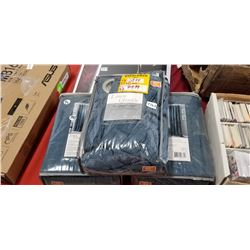162 - Shelf One
CDC Auctions
Shelf One
Bidding Has Concluded

Sold to R*****t for (20.00 + 2.60) x 1 = 22.60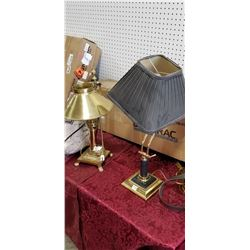170 - Shelf One
CDC Auctions
Shelf One
Bidding Has Concluded

Sold to f***r for (10.00 + 1.30) x 1 = 11.30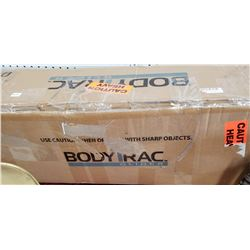171 - Shelf One
CDC Auctions
Shelf One
Bidding Has Concluded

Sold to c********a.. for (32.50 + 4.23) x 1 = 36.73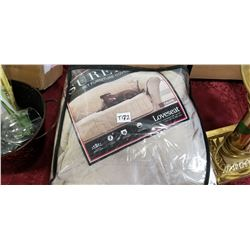172 - Shelf One
CDC Auctions
Shelf One
Bidding Has Concluded

Sold to M*******0 for (10.00 + 1.30) x 1 = 11.30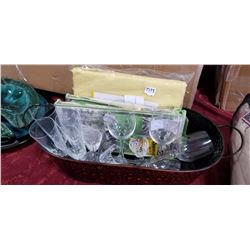173 - Shelf One
CDC Auctions
Shelf One
Bidding Has Concluded

Sold to f***r for (10.00 + 1.30) x 1 = 11.30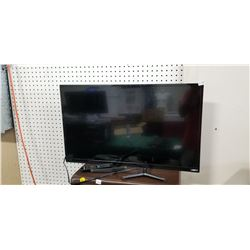178 - Shelf Two
CDC Auctions
Shelf Two
Bidding Has Concluded

Sold to f***r for (75.00 + 9.75) x 1 = 84.75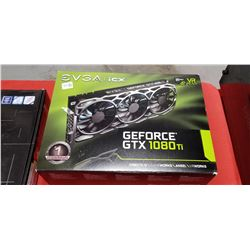180 - Shelf One
CDC Auctions
Shelf One
Bidding Has Concluded

Sold to f***r for (625.00 + 81.25) x 1 = 706.25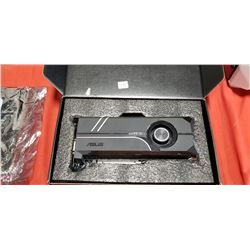181 - Shelf One
CDC Auctions
Shelf One
Bidding Has Concluded

Sold to f***r for (150.00 + 19.50) x 1 = 169.50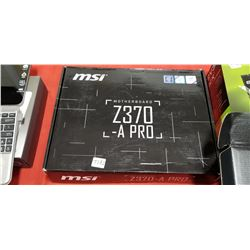182 - Shelf One
CDC Auctions
Shelf One
Bidding Has Concluded

Sold to S********r.. for (40.00 + 5.20) x 1 = 45.20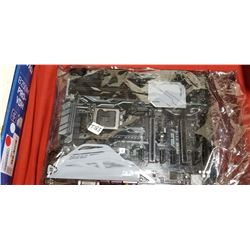183 - Shelf One
CDC Auctions
Shelf One
Bidding Has Concluded

Sold to l*****0 for (70.00 + 9.10) x 1 = 79.10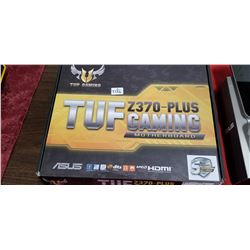186 - Shelf One
CDC Auctions
Shelf One
Bidding Has Concluded

Sold to S********r.. for (30.00 + 3.90) x 1 = 33.90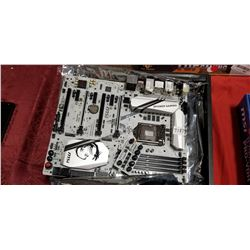187 - Shelf One
CDC Auctions
Shelf One
Bidding Has Concluded

Sold to f***r for (32.50 + 4.23) x 1 = 36.73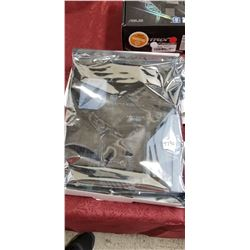190 - Shelf One
CDC Auctions
Shelf One
Bidding Has Concluded

Sold to f***r for (22.50 + 2.93) x 1 = 25.43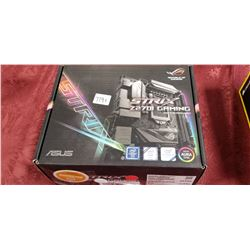191 - Shelf One
CDC Auctions
Shelf One
Bidding Has Concluded

Sold to L*********7 for (75.00 + 9.75) x 1 = 84.75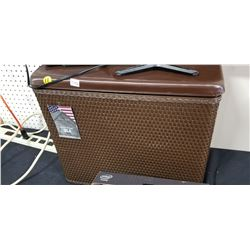192 - Shelf One
CDC Auctions
Shelf One
Bidding Has Concluded

Sold to f***r for (10.00 + 1.30) x 1 = 11.30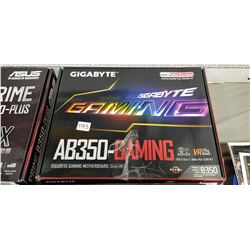193 - Shelf One
CDC Auctions
Shelf One
Bidding Has Concluded

Sold to k********z for (17.50 + 2.28) x 1 = 19.78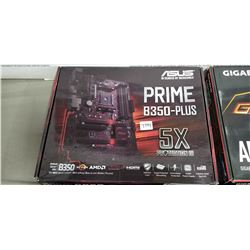194 - Shelf One
CDC Auctions
Shelf One
Bidding Has Concluded

Sold to k********z for (20.00 + 2.60) x 1 = 22.60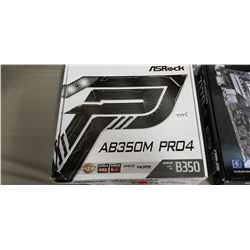195 - Shelf One
CDC Auctions
Shelf One
Bidding Has Concluded

Sold to k********z for (10.00 + 1.30) x 1 = 11.30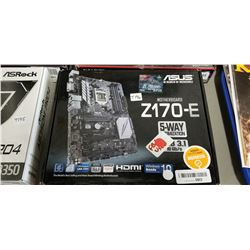196 - Shelf Two
CDC Auctions
Shelf Two
Bidding Has Concluded

Sold to f***r for (22.50 + 2.93) x 1 = 25.43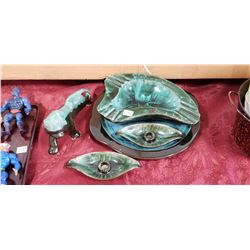197 - Shelf One
CDC Auctions
Shelf One
Bidding Has Concluded

Sold to f***r for (10.00 + 1.30) x 1 = 11.30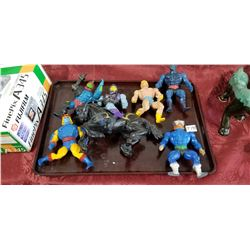198 - Shelf One
CDC Auctions
Shelf One
Bidding Has Concluded

Sold to f***r for (17.50 + 2.28) x 1 = 19.78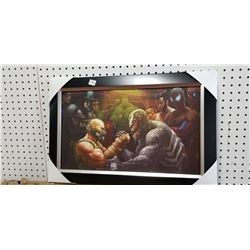200 - Shelf One
CDC Auctions
Shelf One
Bidding Has Concluded

Sold to f***r for (25.00 + 3.25) x 1 = 28.25